Courses

# Test: Valence Bond Theory, Effective Atomic Number And Magnetic Properties

## 25 Questions MCQ Test Chemistry Class 12 | Test: Valence Bond Theory, Effective Atomic Number And Magnetic Properties

Description
This mock test of Test: Valence Bond Theory, Effective Atomic Number And Magnetic Properties for JEE helps you for every JEE entrance exam. This contains 25 Multiple Choice Questions for JEE Test: Valence Bond Theory, Effective Atomic Number And Magnetic Properties (mcq) to study with solutions a complete question bank. The solved questions answers in this Test: Valence Bond Theory, Effective Atomic Number And Magnetic Properties quiz give you a good mix of easy questions and tough questions. JEE students definitely take this Test: Valence Bond Theory, Effective Atomic Number And Magnetic Properties exercise for a better result in the exam. You can find other Test: Valence Bond Theory, Effective Atomic Number And Magnetic Properties extra questions, long questions & short questions for JEE on EduRev as well by searching above.
QUESTION: 1

### Only One Option Correct Type Direction (Q. Nos. 1- 10) This section contains 10 multiple choice questions. Each question has four choices (a), (b), (c) and (d), out of which ONLY ONE is correct. Q.  Which one of the following is wrongly matched?

Solution:

Due to strong ligand, this is inner orbital complex and the hybridisation of Fe is d2sp3.

QUESTION: 2

### In which of the following the central atom has sp3d2-hybridisation?

Solution:

Since, F- ion is a weak ligand hence, pairing of electrons does not occur and outer orbital complex is formed in [CoF6]3-.

QUESTION: 3

### Which is the diamagnetic?

Solution:

[Ni(CN)4]2- in this, Ni has dsp2 hybridisation with square planar geometry and it is diamagnetic.

QUESTION: 4

A magnetic moment of 1.73 BM will be shown by one among following.

Solution:

A magnetic moment of 1.73 BM corresponds to one unpaired electron. Among the given, (a) and (b) are diamagnetic and (c) is paramagnetic with 3 unpaired electrons (3d7 configuration).

QUESTION: 5

Which one of the following complex species does not involve inner orbital hybridisation?

Solution:

F- ion is weak ligand hence, pairing of electrons does not occur and outer orbital complex is formed in [CoF6]3-.

QUESTION: 6

Which of the following complex has zero magnetic moment (spin only)?

Solution:

The potassium ferrocyanide, K4 [Fe(CN)6] due to strong ligand pairing of electrons occur and it has no unpaired electrons.

QUESTION: 7

The number of unpaired electrons calculated in [Co(NH3)6]3+ and [CoF6]3- are

Solution:

In [Co(NH3)6]3+ due to pairing cobalt configuration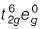and it is diamagnetic.
In [CoF6]3- , F- being weak ligand pairing does not occur and Co3+ ion has configuration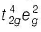. It has 4 unpaired electrons.

QUESTION: 8

The tetrachloro complexes of Ni(ll) and Pd(ll) respectively are (atomic number of Ni and Pd are 28 and 46 respectively).

Solution:

ln [NiCI4]2- , Ni has sp3 hybridisation with 2 unpaired electrons, i.e. paramagnetic.
In [PdCI4]2- Pd has dsp2 hybridisation and it has no unpaired electrons, i.e. diamagnetic.

QUESTION: 9

Which one of the following is an inner orbital complex as well as diamagnetic in behaviour? (Atomic number, Zn = 30, Cr= 24, Co = 27, Ni = 28)

Solution:

Zn and Ni always give outer orbital complexes with coordination number 6.
[Co(NH3)6]3+ is diamagnetic complex.
[Cr(NH3)6]3+ is param agnetic with 3d3 configuration.

QUESTION: 10

The pair of compounds having the same hybridisation for the central atom is

Solution:

[Co(NH3)6]3+ : For this complex action the 6 valance electrons of cobalt will occupy the 3d orbital, whereas the 6 ligands will occupy 3d,4s and 4p orbitals in d2sp3 hybridisation.

[Co(H2O)6]3+ : This intermixing is based on quantum mechanics. Transition metals may exhibit d2sp3 hybridization where the d orbitals are from the 3d and the s and p orbitals are the 4s and 3d. it acts as strong ligand when metal ion is in +3 oxidation for metals cobalt.

*Multiple options can be correct
QUESTION: 11

One or More than One Options Correct Type

Direction (Q. Nos. 11-15) This section contains 5 multiple choice questions. Each question has four choices (a), (b), (c) and (d), out of which ONE or MORE THAN ONE are correct.

Q.

The formation of complex which involves d-orbitals of outer shell are called

Solution:

The complex which involves d-orbitals of outer shell are called high spin complex as well as outer orbital complex.

*Multiple options can be correct
QUESTION: 12

sp3-hybridisation is found in

Solution:

[ZnCI4]2-, [CuCI4]2- and [Ni(CO)4] have sp3 hybridisation and all three contains weak field ligands.

*Multiple options can be correct
QUESTION: 13

Regarding the complex [Co(H2O)6]2- , correct statement(s) is/are

Solution:

Since, F- ion is weak ligand it forms outer orbital complex arid it has 3d7 configuration with 3 unpaired electrons.

*Multiple options can be correct
QUESTION: 14

For which the EAN value is equal to the atomic number of a noble gas?

Solution:

K2[Hgl4] In this EAN = 80 - 2 + 8 = 86 (Equal to noble gas, radon).
[Pd(NH3)CI2] In this EAN = 46 - 2 + 8 = 52 (Not equal to noble gas, xenon)
[Cdl4]2- In this EAN = 48 - 2 + 8 = 54 (Equal to noble gas, xenon)
Co2(CO)8 In this EAN = Electrons from Co-atom (27) + electrons from 4 CO molecules (8) + one electron from Co— Co bond (1) = 36 (Equal to noble gas krypton)

*Multiple options can be correct
QUESTION: 15

[Fe(H2O)6]2+ and [Fe(CN)6]4- differ in

Solution:

[Fe(H2O)6]2+ and [Fe(CN)6]4- have different hybridisation, magnetic moment and colour also.

QUESTION: 16

Comprehension Type

Direction (Q. Nos. 16 and 17) This section contains a paragraph, describing theory, experiments, data, etc. Two questions related to the paragraph have been given. Each question has only one correct answer among the four given options (a), (b), (c) and (d).

Passage

When crystals of CuSO4 . 4NH3 are dissolved in water, there is hardly any evidence for the presence of Cu2+ ions or ammonia molecules. A new ion [Cu(NH3)4]2+ is furnished in which ammonia molecules are directly linked with the metal ion. Similarly, aqueous solution of Fe(CN)2 .4KCNdoes not give tests of Fe2+ and CN- ions but give test for new ion [Fe(CN)6]4- called ferrocyanide ion.

Q.

The hybridisation and geometry and magnetic property of [Cu(NH3)4]2+ ion are

Solution:

Cu(Z = 29) copper configuration (3d104s1)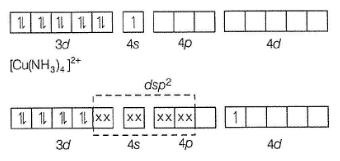Hence, [Cu(NH3)4]2+ has square planar geometry and 1 unpaired electron in Ad orbital which is transferred from 3d orbital with magnetic moment 1.73 BM.

QUESTION: 17

When crystals of CuSO4 . 4NH3 are dissolved in water, there is hardly any evidence for the presence of Cu2+ ions or ammonia molecules. A new ion [Cu(NH3)4]2+ is furnished in which ammonia molecules are directly linked with the metal ion. Similarly, aqueous solution of Fe(CN)2 .4KCNdoes not give tests of Fe2+ and CN- ions but give test for new ion [Fe(CN)6]4- called ferrocyanide ion.

Q.

The hybridisation, geometry and magnetic property of [Fe(CN)6]4- are

Solution:

It is inner orbital complex, diamagnetic.

QUESTION: 18

Matching List Type

Direction (Q. Nos. 18 and 19) Choices for the correct combination of elements from Column I and Column II are given as options (a), (b), (c) and (d) out of which one is correct.

Q.

Match the Column I with Column II and mark the correct option from the codes given bleow.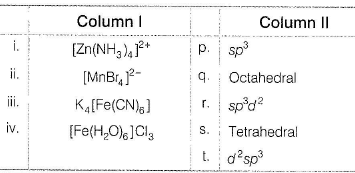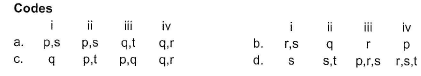Solution:

(i) → (p.s), (ii) → (p,s), (iii) → (q,t), (iv) → (q.r)

QUESTION: 19

Match the Column I with Column il and mark the correct option from the codes given bleow.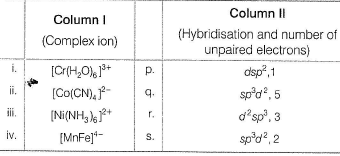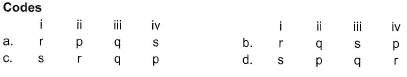Solution:

A → (iii) (b) B → (i) (c) C → (iv) (d) D → (ii)

a) Six empty orbitals (two d, one s and three p) are available, so whether the ligand is weak (H2​O)  the six orbitals hybridize to give six equivalent d2sp3 hybrid orbitals. Hence, in  [Cr(H2​O)6​]3+  ions chromium is in a state of d2sp3 hybridization.b)  CN is a strong ligand it makes the unpaired electrons of cobalt to pair up and occupies the space.

• The ligands occupy one d orbital, one s orbital and 2 p orbitals. Thus the hybridisation is “dsp2” with “square planar” geometry.
• It has 1 unpaired electron.

c) In [Ni(NH3)6]2+, Ni is in +2 state and has configuration 3d8. In presence of NH3, the 3d electrons do not pair up. The hybridization is sp3d2 forming an outer orbital complex.

It has been found that the complex has two unpaired electrons.

*Answer can only contain numeric values
QUESTION: 20

One Integer Value Correct Type

Direction (Q. Nos. 20-24) This section contains 5 questions. When worked out will result in an integer from 0 to 9 (both inclusive).

Q.

How many of the following species obey Sidgwick EAN rule?

K3[Fe(CN)6], [Ru(CO)5], [Cr(NH3)6] 3+, [Co(NH3)6]3+, [Ni(NH3)6]2+, [Fe(CO)5],[W(CO)6]

Solution: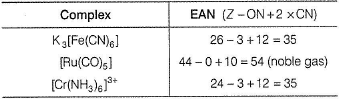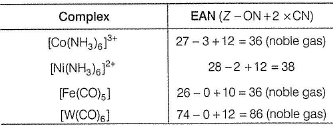*Answer can only contain numeric values
QUESTION: 21

How many of the following are diamagnetic?

[Zn(OH)4]2-,[Ni(NH3)6]2+, K4[Fe(CN)6], K3[Fe(CN)6], [Cu(NH3)4]2+,[PdBr4]2-, [Ni(CO)4], [CoF6] 3-

Solution:

Diamagnetic are
[Zn(OH)4]2-, K4[Fe(CN)6], [PdBr4]2-,[Ni(CO)4]

*Answer can only contain numeric values
QUESTION: 22

The number of unpaired electrons in the complex [Mn(acac)3] is

(Atomic number of Mn= 25)

Solution:

In this, Mn has +3 oxidation state. Mn3+ configuration = [Ar]3d4. This forms outer complex. Hence, unpaired electrons are 4.

*Answer can only contain numeric values
QUESTION: 23

The magnetic moment of [Ru(H2O)6]2+ corresponds to the presence of ...... unpaired electrons.

Solution:

R = [Kr]4d7 5s1 (in ground state)
In Ru[2+] => 4d6
=> (t2g)6 (eg)0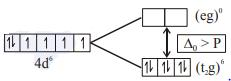Hence, no unpaired electron. Therefore, correct answer is zero.

*Answer can only contain numeric values
QUESTION: 24

The number of unpaired electrons in [Fe(dipy)3]2+ are

Solution:

In [Fe(dipy)3]2+ complex ion, Fe has 3d6 configuration. Due to pairing, this has no unpaired electrons.

QUESTION: 25

Statement Type

Direction (Q. No. 25) This section is based on Statement I and Statement II. Select the correct answer from the codes given below.

Q.

Statement I : Both [Ni(CN)4]2- and [NiCI4]2- have same shape and same magnetic behaviour.

Statement II : Both are square planar and diamagnetic

Solution:

Statement I [Ni(CN)4]2- is square planar and diamagnetic whereas [NiCI4]2- is tetrahedral and paramagnetic .
Statement II [Ni(CN)4]2- is square planar while [NiCI4]2- is tetrahedral.